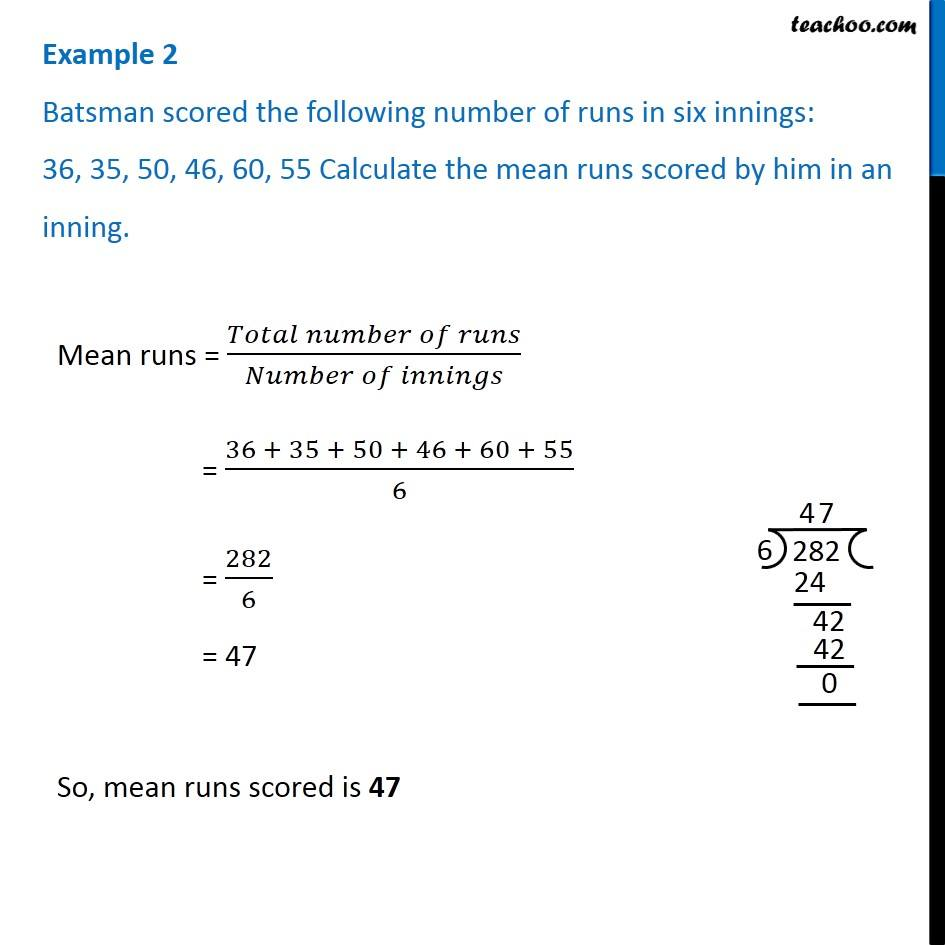Mean

Chapter 3 Class 7 Data Handling
Concept wiseIntroducing your new favourite teacher - Teachoo Black, at only ₹83 per month

### Transcript

Example 2 Batsman scored the following number of runs in six innings: 36, 35, 50, 46, 60, 55 Calculate the mean runs scored by him in an inning. Mean runs = (𝑇𝑜𝑡𝑎𝑙 𝑛𝑢𝑚𝑏𝑒𝑟 𝑜𝑓 𝑟𝑢𝑛𝑠)/(𝑁𝑢𝑚𝑏𝑒𝑟 𝑜𝑓 𝑖𝑛𝑛𝑖𝑛𝑔𝑠) = (36 + 35 + 50 + 46 + 60 + 55)/6 = 282/6 = 47 So, mean runs scored is 47